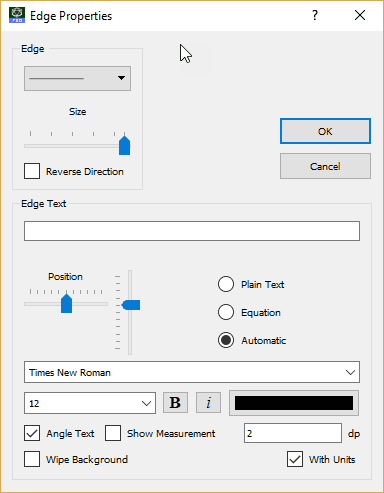﻿ Triangles

# Triangles

Top  Previous  NextDrawing Triangles

# General Triangle Tool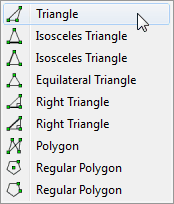The general triangle tool allows you to draw any triangle.

Right-clicking on one of the sides of a triangle will allow you adjust the sides and angles of your triangle. You can also add marks and text to the edge.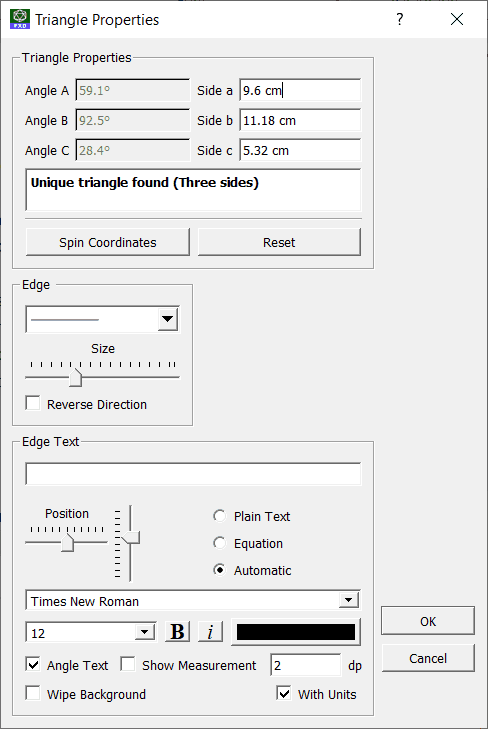The triangle properties dynamically determine if you have entered sufficient information to define a unique triangle. In this example, we have removed the length of the first side and the remaining information is insufficient to define the triangle. FX Draw then allows you to enter other information (for example angles) until such time as a unique (and valid) triangle is defined.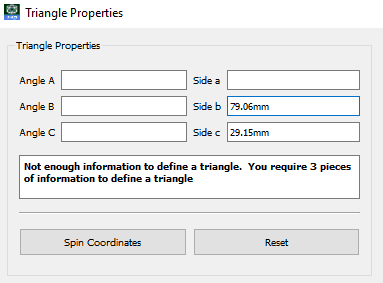In this example we have entered three sides which cannot form a triangle, so FX Draw has not locked off the other options.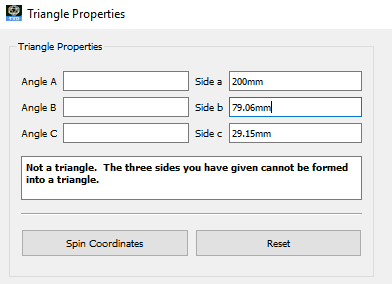Available Parameters for Triangles

\$area

\$aalt

\$balt                Altitudes

\$calt

\$perimeter

\$a

\$b                Angle sizes

\$c

\$sa

\$sb                Side lengths

\$sc

# Isosceles Triangles 1 & 2

The isosceles triangles tools provide slightly different ways of drawing isosceles triangles.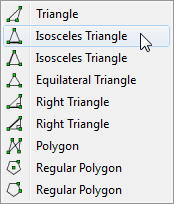When using the first isosceles triangle tool, the first line you draw is the base of the isosceles triangle.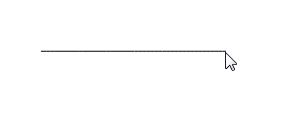When using the second isosceles triangle tool, the first line draw is one of the two congruent sides.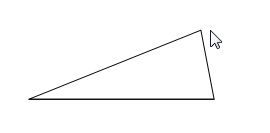Right-clicking on one of the sides of a triangle will allow you to add marks and text to the edges of your triangle. You also have the option to automatically mark the equal length sides.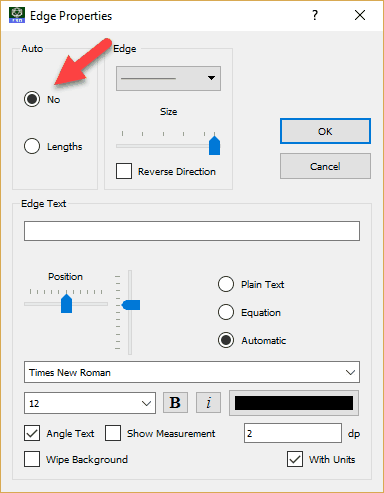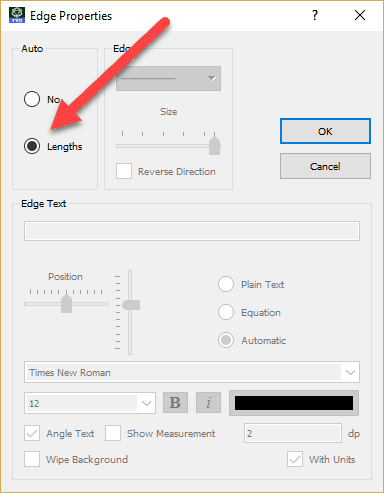# Equilateral Triangle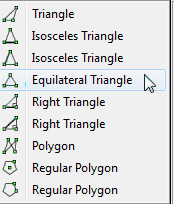The equilateral triangle tool allows you to quickly draw equilateral triangles.

Right-clicking on one of the sides of a triangle will allow you to add marks and text to the edges of your triangle. You also have the option to automatically mark the equal length sides.# Right Triangles 1 & 2

The right triangles tools provide slightly different ways of drawing right triangles.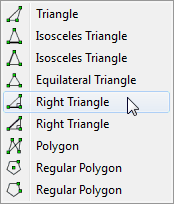When using the first right triangle tool, the first line you draw is one of the shorter sides of the right triangle.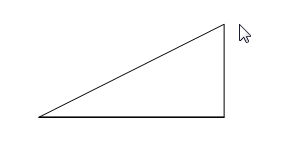When using the second right triangle tool, the first line draw is the hypotenuse.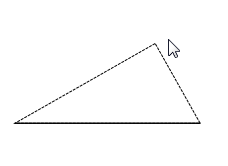Right-clicking on one of the sides of a triangle will allow you to add marks to the middle of the line.